Paper Cup
 What is a Paper Cup? Folding of a Paper Cup Variations Some Mathematics Paper Cup on the Internet .
 To my Main Page    "Mathematische Basteleien"

What is a Paper Cup?
 ...... A paper cup is a cup which you can fold from a sheet of paper. If the paper is not printed inside and sturdy, you can even drink from it.
If the paper cup is large enough, you can turn it and use it as a hat.

Folding of a Paper Cup top
1
 ...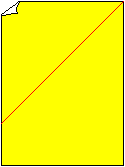... Take a sheet of paper of the size A4 (8 1/2" x 11"). Paper used for ink jet printers will do.  Fold the upper half down on the red line.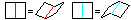2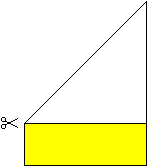... Cut off the lower strip.3
 ...... The result is a square.4
 ...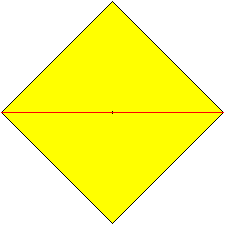... Fold the upper triangle downwards on the red line.5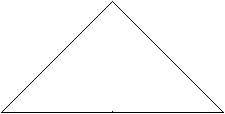The result is a isosceles, right-angled triangle.6Fold the left corner upwards on the red line. Mind, that you get a new isosceles, right-angled triangle (grey) and that its basic side is parallel to the basic side of the whole triangle.7It must look like this.8Fold the right corner upwards on the red line.9
 ...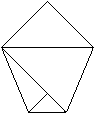... It must look like this.10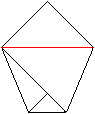Fold the upper triangle downwards on the red line.11It must look like this. There is a second triangle.12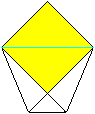Fold this triangle backwards.13.....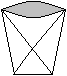....... Open it. The paper cup is finished. It has no stand.Variation   top
 ...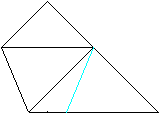... After step 1 to 7 fold the left triangle backwards at the blue line.  Both triangles, in front and on the rear side, form pockets. You can put in the upper triangles. You can cut them, so that they fit. Then the paper cup looks better.

Some Mathematics  top
If you unfold the paper, you can recognize the shapes of the cup in the shape of a trapezoid
 ...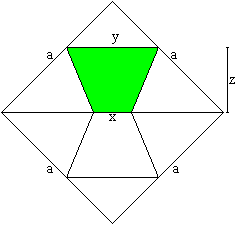... How can you calculate the measurements of the cup, when the side of the square is given as a?  A trapezoid is given by the parallel sides x, y and the height z for instance.

If you like to calculate yourself, stop reading further.If you fold on the red line, you lay the left yellow triangle on the right. Thus the yellow quadrilateral has the sides b and b' twice. Both diagonals are perpendicular to each other. Therefore the quadrilateral is a rhombus and all sides have the same length. The upper triangle is isosceles and right-angled. Its two sides have the length sqr(2)/2*b. If the side of the starting square is a (green), there is a=b + sqrt(2)/2*b. Then b is  [2-sqrt(2)]*a.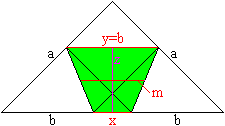The trapezoid, which develops, is given by x, y and z. There is:  x=sqrt(a)-2b = [3*sqrt(2)-4]*a  y=b=[2-sqr(2)]*a z=[sqr(2)/2]*a - b/2 = [sqr(2)-1]*aThe median is m=(x+y)/2=[sqrt(2)-1]*a =z. Thus the median and the height of the trapezoid have the same lengths.

By the way the upper corners of the trapezoid devide the side a in the ratio 1:sqrt(2). This is the ratio of  the format A.

Paper Cup on the Internet   top

German:

Andreas Bauer

Besser Basteln
Origami Becher

Labbé (zzzebra)
Trinkbecher aus Papier

Mathekiste
Symmetrie und Papierfalten

origami.ch
Trinkbecher

English:

Kids Web Japan
Let's Make Origami!

ViewDo LLC
Paper cup (Video)

Feedback: Email address on my main page